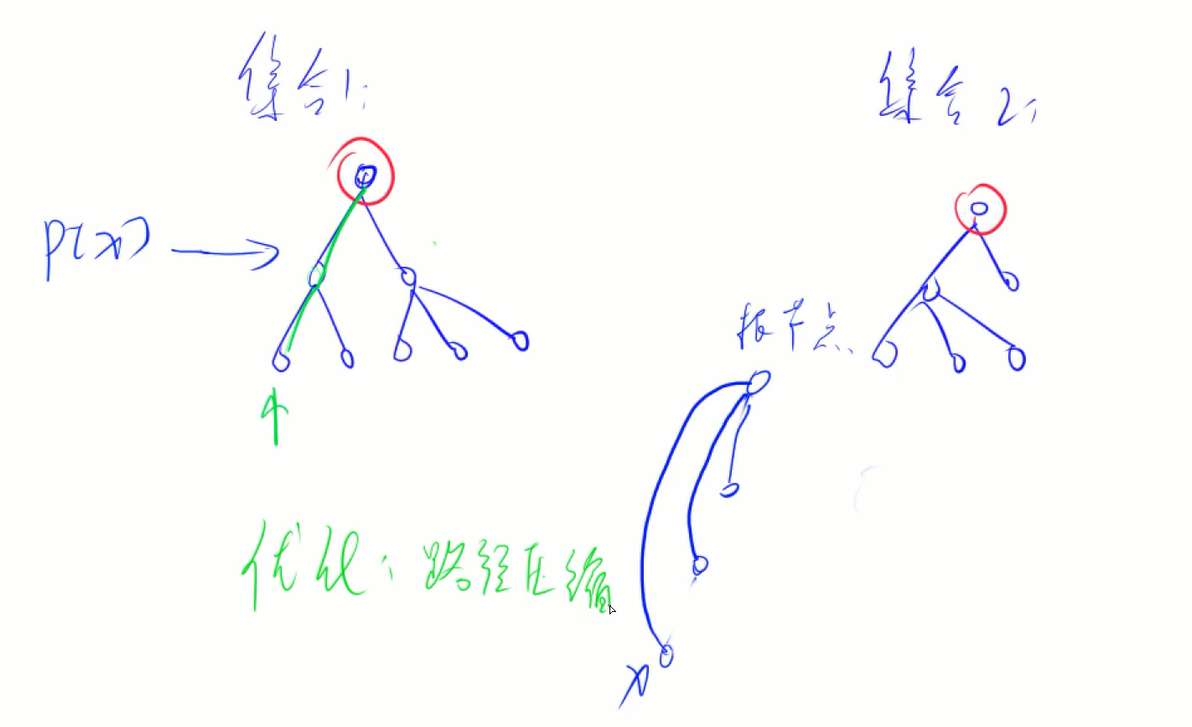## Catalog# 【简单】合并集合（并查集）

## Henry • June 11, 2021 • Read: 1015 • 学习笔记,算法,算法&题库

1. “M a b”，将编号为 $a$ 和 $b$ 的两个数所在的集合合并，如果两个数已经在一个集合中，则忽略这个操作；
2. “Q a b”，询问编号为 $a$ 和 $b$ 的两个数是否在同一集合中。

#### 数据范围

$\rm{1} \le n,m \le {10^5}$

#### 输入样例

4 5
M 1 2
M 3 4
Q 1 2
Q 1 3
Q 3 4

#### 输出样例

Yes
No
Yes

#### 题解

（并查集） 数据结构

1. 将两个集合合并
2. 询问两个元素是否在一个集合当中#### C++ 代码

#include <iostream>

using namespace std;

const int N = 100010;

int n, m;
int p[N];

int find(int x) //返回x所在集根节点 + 路径压缩优化
{
if (p[x] != x)
p[x] = find(p[x]);
return p[x];
}

int main()
{
scanf("%d%d", &n, &m);
for (int i = 1; i <= n; i++)
p[i] = i;//初始化，每个数各自在一个集合
while (m--)
{
char op;
int a, b;
cin >> op >> a >> b;
if(op == 'M')
p[find(a)] = find(b);
else
find(a) == find(b) ? puts("Yes") : puts("No");
}
return 0;
}